# Chapter 7 Problem Solving and Algorithms Problem Solving

• Slides: 73Chapter 7 Problem Solving and AlgorithmsProblem Solving Problem solving The act of finding a solution to a perplexing, distressing, vexing, or unsettled question How do you define problem solving? 2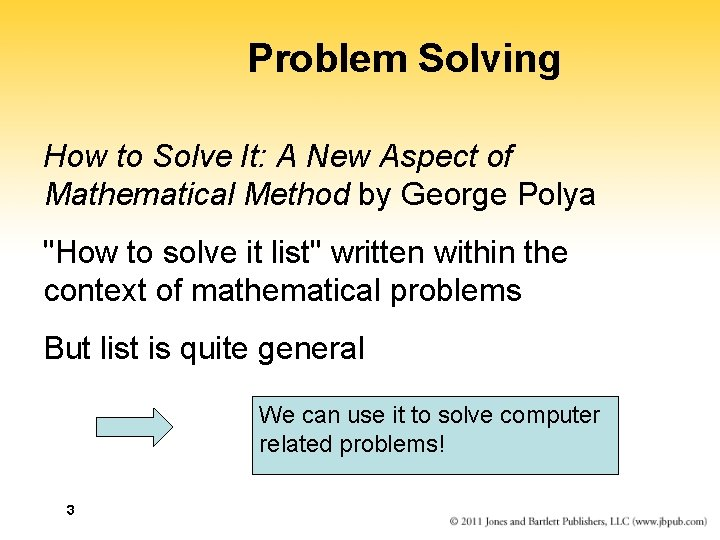Problem Solving How to Solve It: A New Aspect of Mathematical Method by George Polya "How to solve it list" written within the context of mathematical problems But list is quite general We can use it to solve computer related problems! 3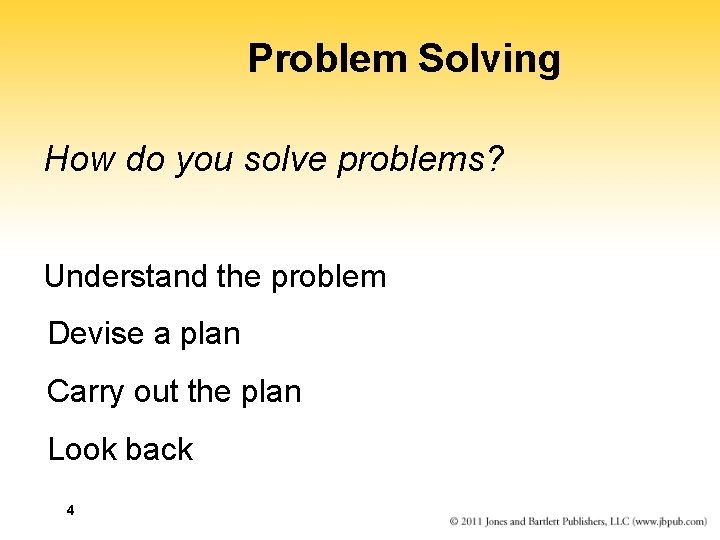Problem Solving How do you solve problems? Understand the problem Devise a plan Carry out the plan Look back 4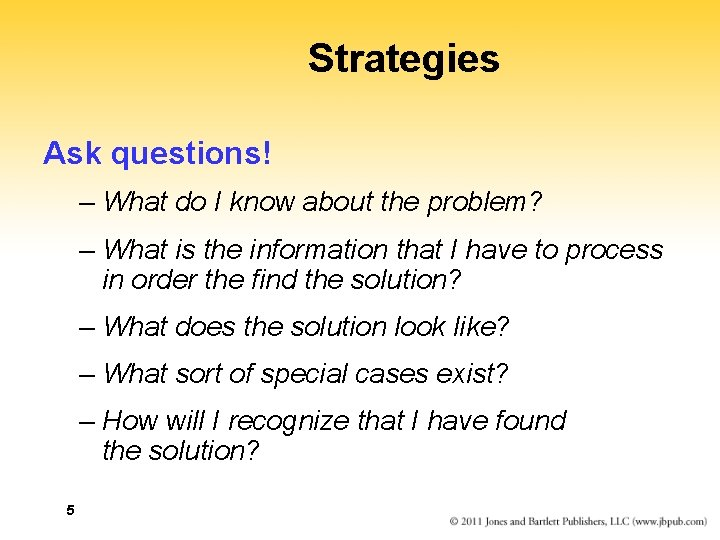Strategies Ask questions! – What do I know about the problem? – What is the information that I have to process in order the find the solution? – What does the solution look like? – What sort of special cases exist? – How will I recognize that I have found the solution? 5Strategies Ask questions! Never reinvent the wheel! Similar problems come up again and again in different guises A good programmer recognizes a task or subtask that has been solved before and plugs in the solution Can you think of two similar problems? 6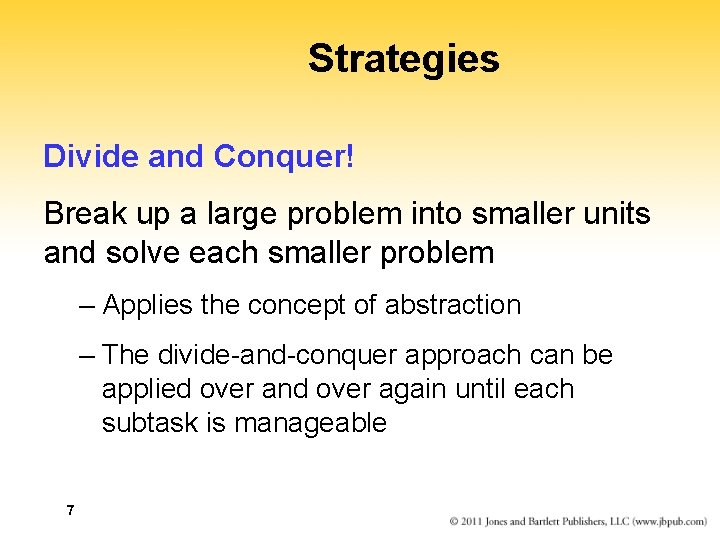Strategies Divide and Conquer! Break up a large problem into smaller units and solve each smaller problem – Applies the concept of abstraction – The divide-and-conquer approach can be applied over and over again until each subtask is manageable 7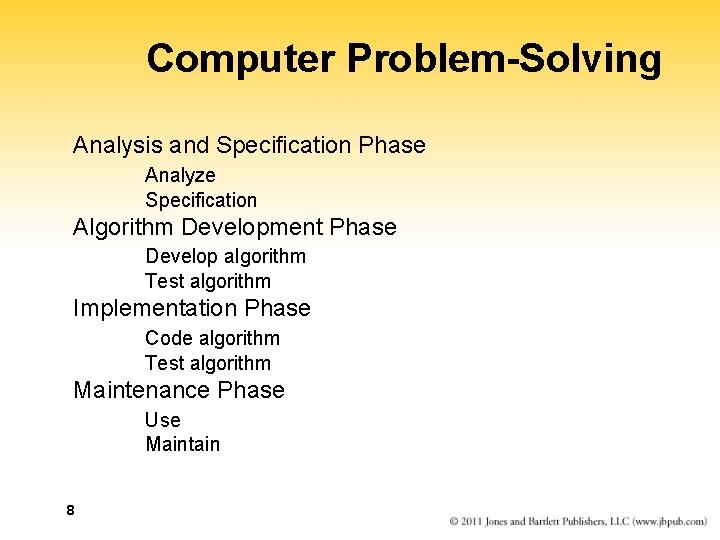Computer Problem-Solving Analysis and Specification Phase Analyze Specification Algorithm Development Phase Develop algorithm Test algorithm Implementation Phase Code algorithm Test algorithm Maintenance Phase Use Maintain 8Phase Interactions 9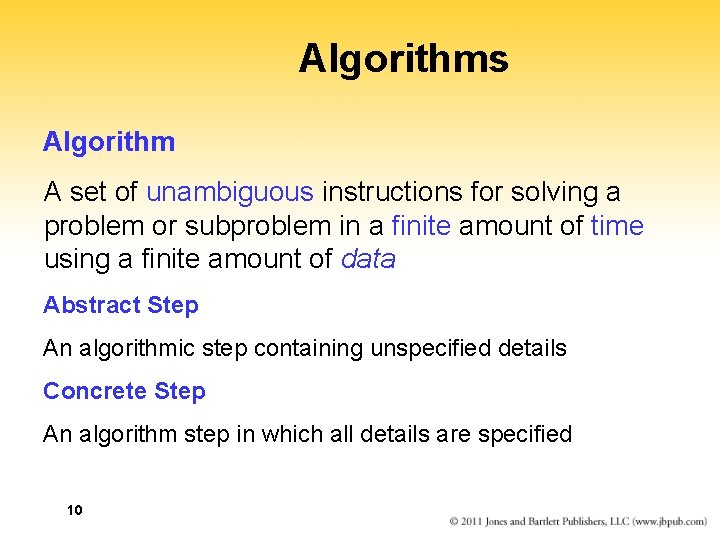Algorithms Algorithm A set of unambiguous instructions for solving a problem or subproblem in a finite amount of time using a finite amount of data Abstract Step An algorithmic step containing unspecified details Concrete Step An algorithm step in which all details are specified 10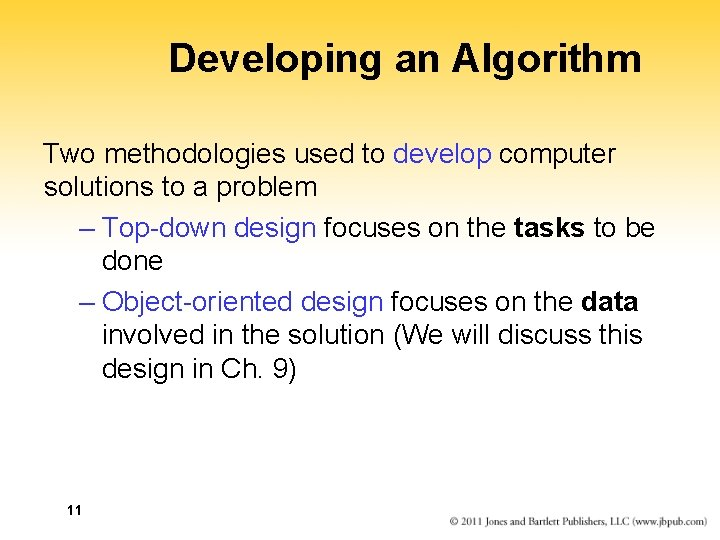Developing an Algorithm Two methodologies used to develop computer solutions to a problem – Top-down design focuses on the tasks to be done – Object-oriented design focuses on the data involved in the solution (We will discuss this design in Ch. 9) 11Summary of Methodology Analyze the Problem Understand the problem!! Develop a plan of attack List the Main Tasks (becomes Main Module) Restate problem as a list of tasks (modules) Give each task a name Write the Remaining Modules Restate each abstract module as a list of tasks Give each task a name Re-sequence and Revise as Necessary Process ends when all steps (modules) are concrete 12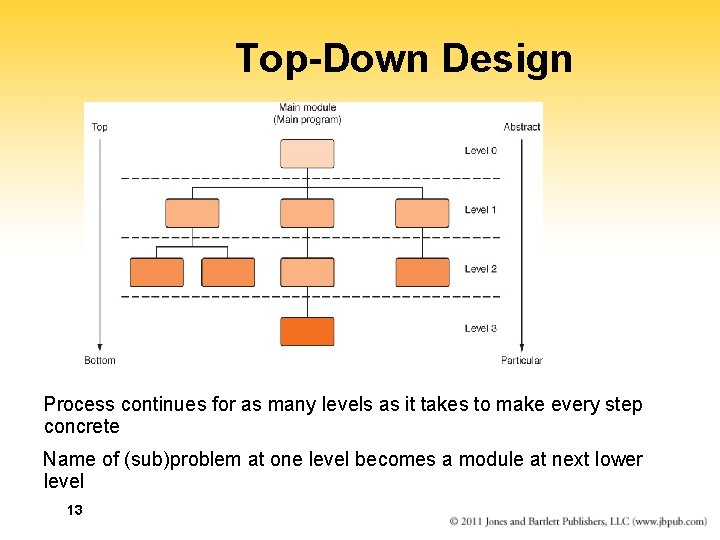Top-Down Design Process continues for as many levels as it takes to make every step concrete Name of (sub)problem at one level becomes a module at next lower level 13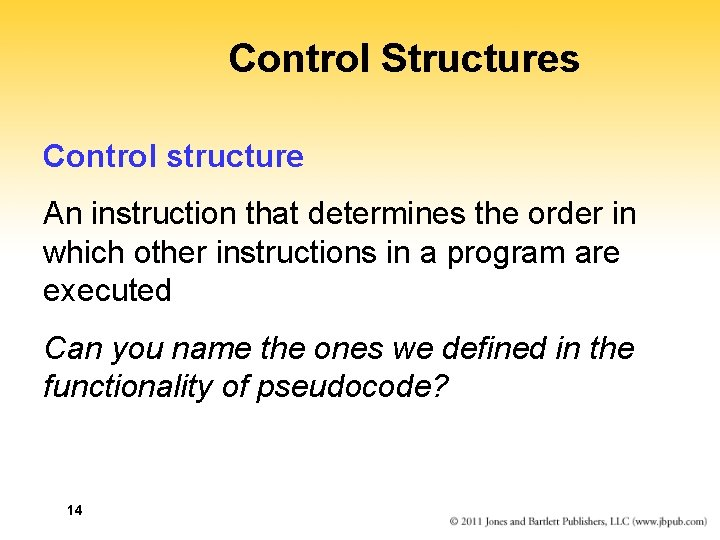Control Structures Control structure An instruction that determines the order in which other instructions in a program are executed Can you name the ones we defined in the functionality of pseudocode? 14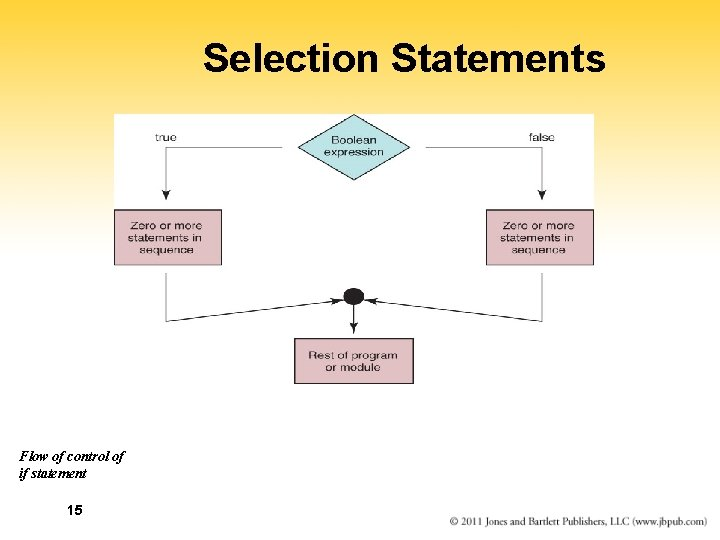Selection Statements Flow of control of if statement 15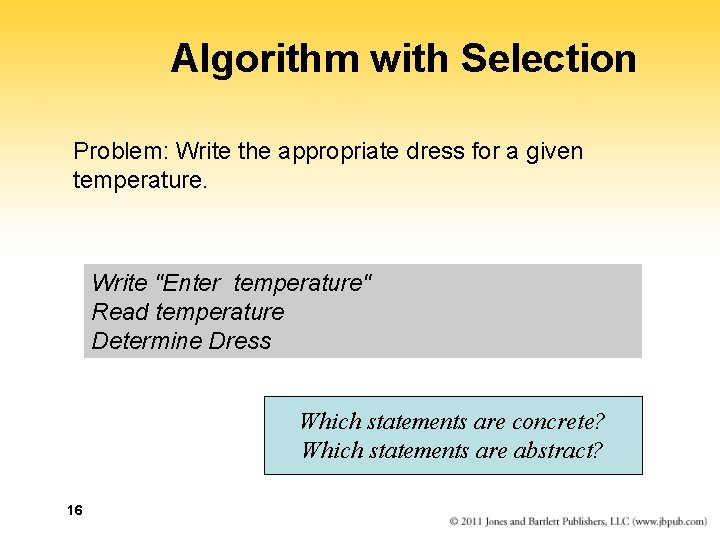Algorithm with Selection Problem: Write the appropriate dress for a given temperature. Write "Enter temperature" Read temperature Determine Dress Which statements are concrete? Which statements are abstract? 16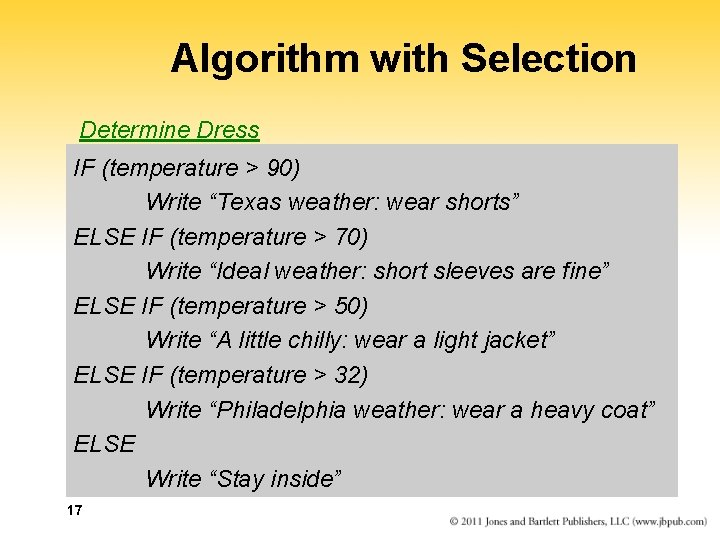Algorithm with Selection Determine Dress IF (temperature > 90) Write “Texas weather: wear shorts” ELSE IF (temperature > 70) Write “Ideal weather: short sleeves are fine” ELSE IF (temperature > 50) Write “A little chilly: wear a light jacket” ELSE IF (temperature > 32) Write “Philadelphia weather: wear a heavy coat” ELSE Write “Stay inside” 17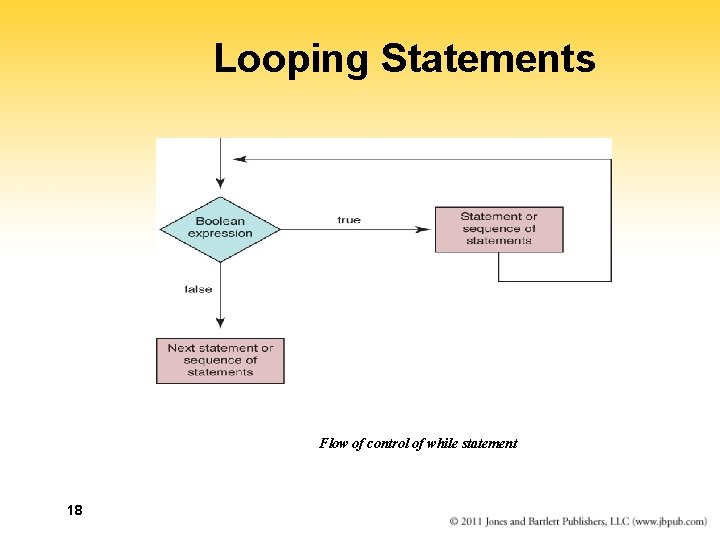Looping Statements Flow of control of while statement 18Looping Statements A count-controlled loop Set sum to 0 Set count to 1 While (count <= limit) Read number Set sum to sum + number Increment count Write "Sum is " + sum 19 Why is it called a count-controlled loop?Looping Statements An event-controlled loop Set sum to 0 Set all. Positive to true WHILE (all. Positive) Read number IF (number > 0) Set sum to sum + number ELSE Set all. Positive to false Write "Sum is " + sum 20 Why is it called an event-conrolled loop? What is the event?Looping Statements Calculate Square Root Read in square Calculate the square root Write out square and the square root Are there any abstract steps? 21Looping Statements Calculate square root Set epsilon to 1 WHILE (epsilon > 0. 001) Calculate new guess Set epsilon to abs(square - guess * guess) Are there any abstract steps? 22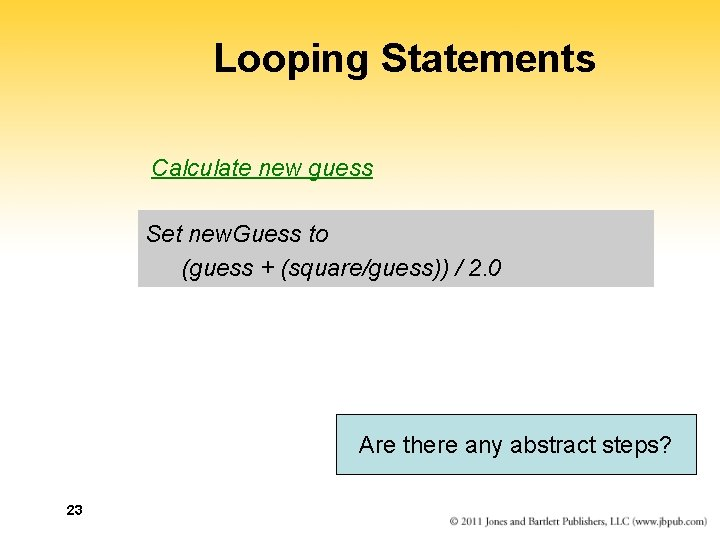Looping Statements Calculate new guess Set new. Guess to (guess + (square/guess)) / 2. 0 Are there any abstract steps? 23Looping Statements Read in square Set guess to square/4 Set epsilon to 1 WHILE (epsilon > 0. 001) Calculate new guess Set epsilon to abs(square - guess * guess) Write out square and the guess 24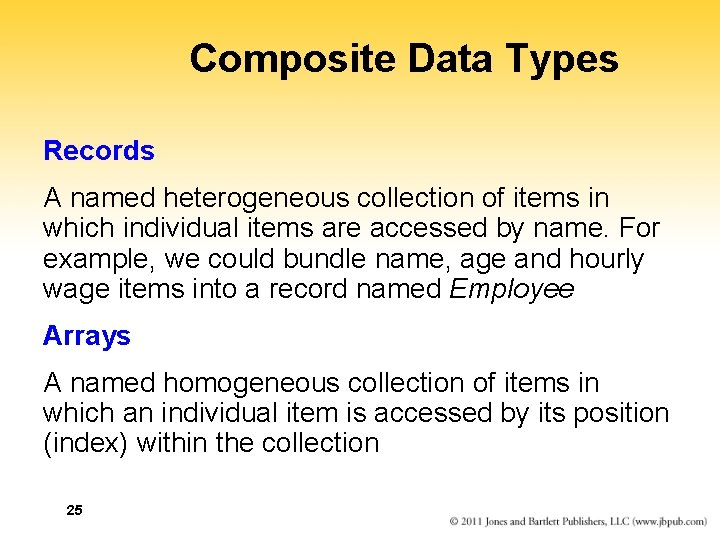Composite Data Types Records A named heterogeneous collection of items in which individual items are accessed by name. For example, we could bundle name, age and hourly wage items into a record named Employee Arrays A named homogeneous collection of items in which an individual item is accessed by its position (index) within the collection 25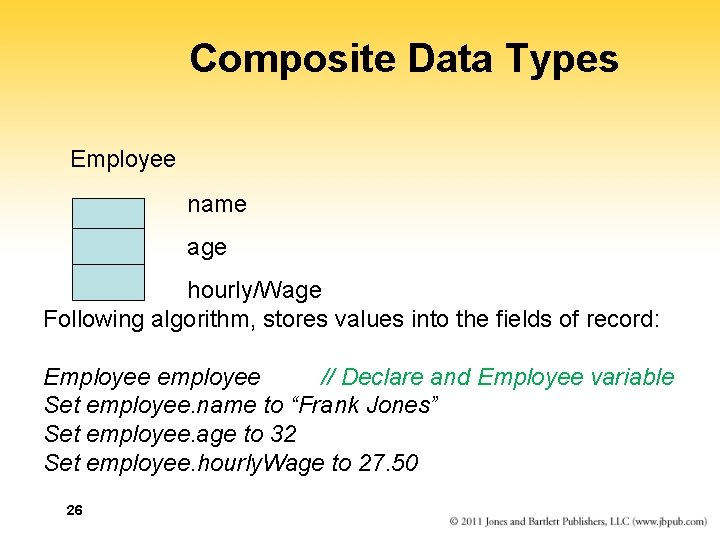Composite Data Types Employee name age hourly/Wage Following algorithm, stores values into the fields of record: Employee employee // Declare and Employee variable Set employee. name to “Frank Jones” Set employee. age to 32 Set employee. hourly. Wage to 27. 50 26Arrays • As data is being read into an array, a counter is updated so that we always know how many data items were stored • If the array is called list, that with which we are working is from list to list [length-1] 27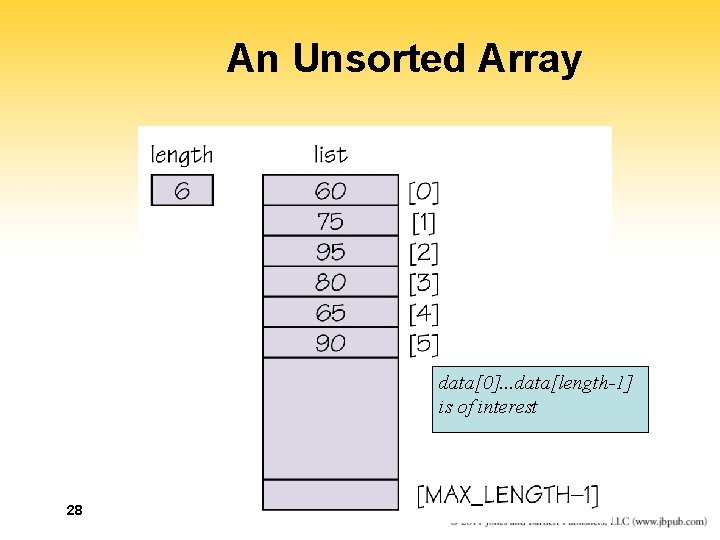An Unsorted Array data. . . data[length-1] is of interest 28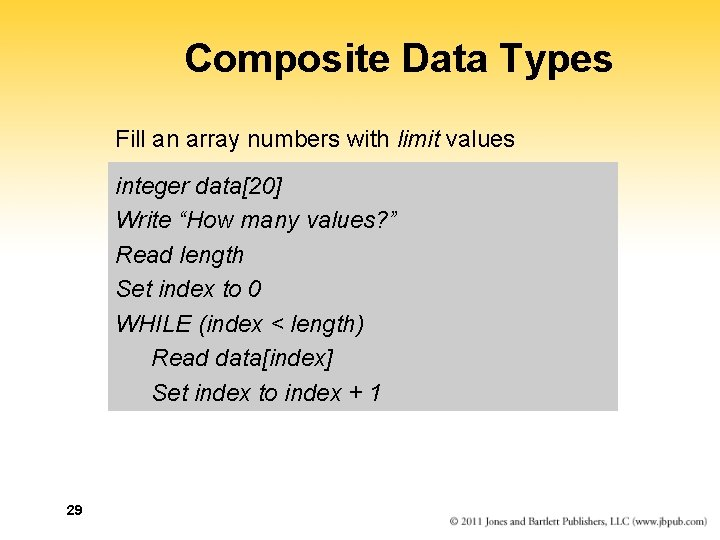Composite Data Types Fill an array numbers with limit values integer data Write “How many values? ” Read length Set index to 0 WHILE (index < length) Read data[index] Set index to index + 1 29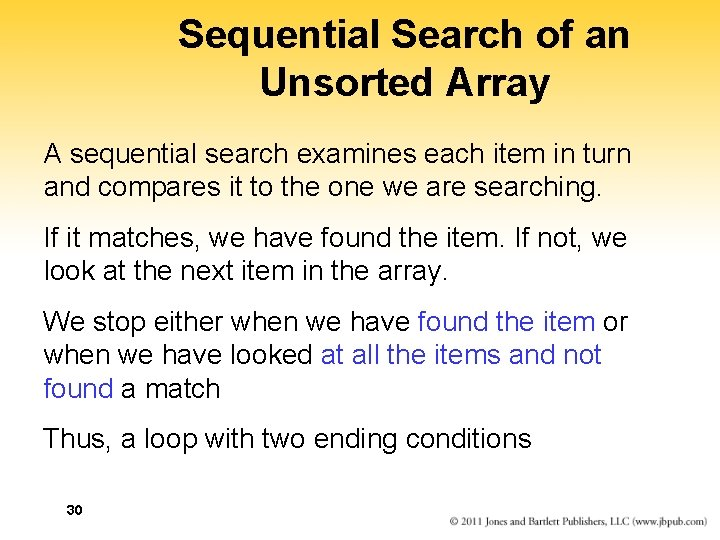Sequential Search of an Unsorted Array A sequential search examines each item in turn and compares it to the one we are searching. If it matches, we have found the item. If not, we look at the next item in the array. We stop either when we have found the item or when we have looked at all the items and not found a match Thus, a loop with two ending conditions 30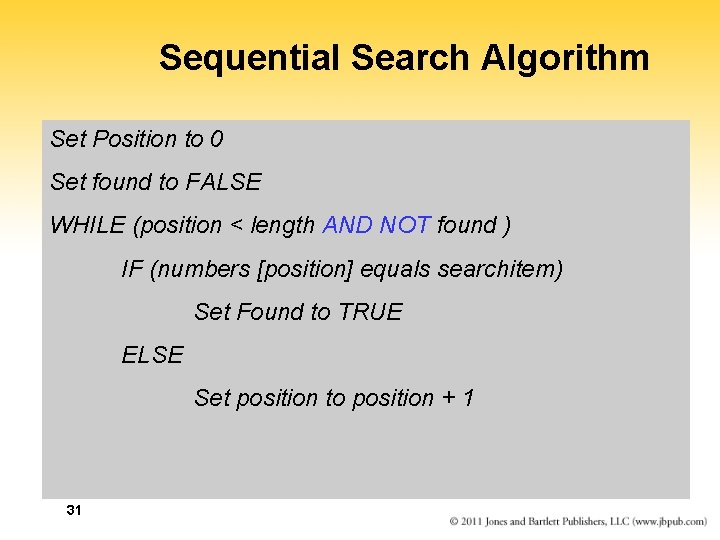Sequential Search Algorithm Set Position to 0 Set found to FALSE WHILE (position < length AND NOT found ) IF (numbers [position] equals searchitem) Set Found to TRUE ELSE Set position to position + 1 31Booleans Boolean Operators A Bolean variable is a location in memory that can contain either true or false Boolean operator AND returns TRUE if both operands are true and FALSE otherwise Boolean operator OR returns TRUE if either operand is true and FALSE otherwise Boolean operator NOT returns TRUE if its operand is false and FALSE if its operand is true 32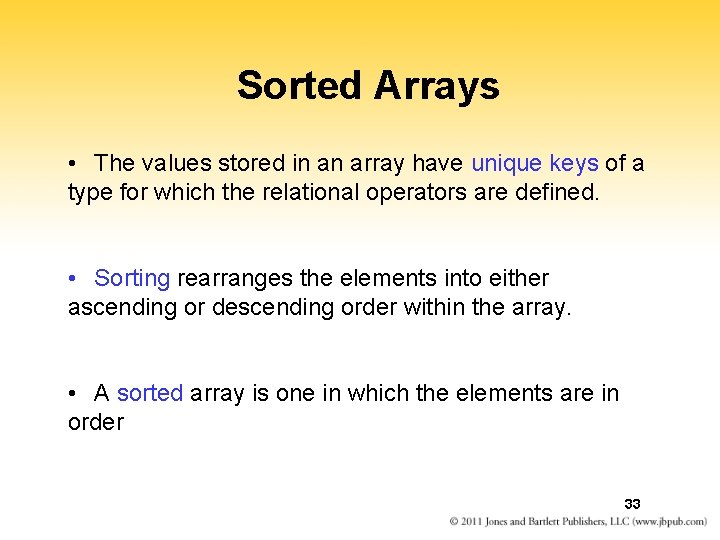Sorted Arrays • The values stored in an array have unique keys of a type for which the relational operators are defined. • Sorting rearranges the elements into either ascending or descending order within the array. • A sorted array is one in which the elements are in order 33Sequential Search in a Sorted Array If items in an array are sorted, we can stop looking when we pass the place where the item would be it were present in the array 34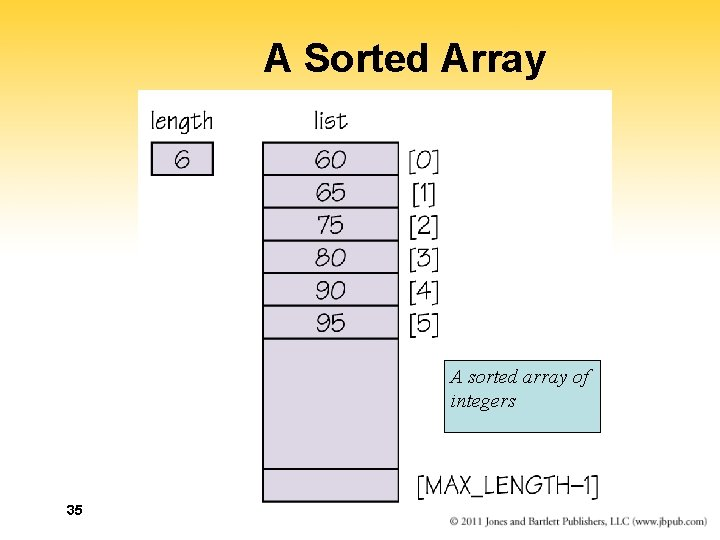A Sorted Array A sorted array of integers 35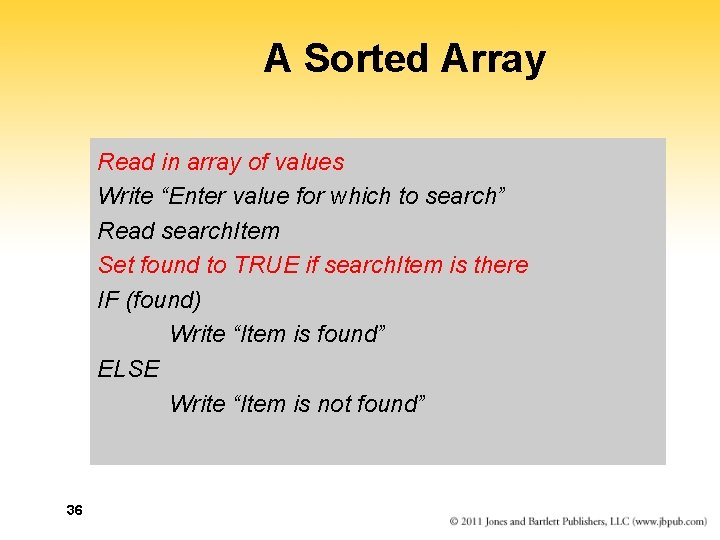A Sorted Array Read in array of values Write “Enter value for which to search” Read search. Item Set found to TRUE if search. Item is there IF (found) Write “Item is found” ELSE Write “Item is not found” 36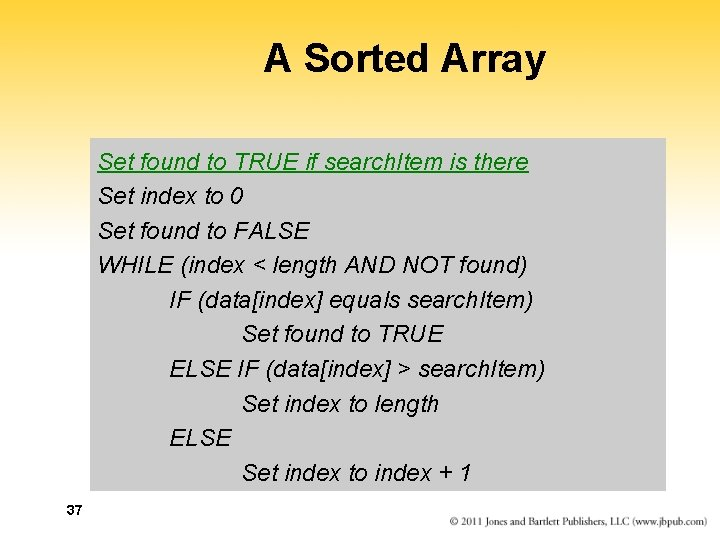A Sorted Array Set found to TRUE if search. Item is there Set index to 0 Set found to FALSE WHILE (index < length AND NOT found) IF (data[index] equals search. Item) Set found to TRUE ELSE IF (data[index] > search. Item) Set index to length ELSE Set index to index + 1 37Binary Search Sequential search Search begins at the beginning of the list and continues until the item is found or the entire list has been searched Binary search (list must be sorted) Search begins at the middle and finds the item or eliminates half of the unexamined items; process is repeated on the half where the item might be Say that again… 38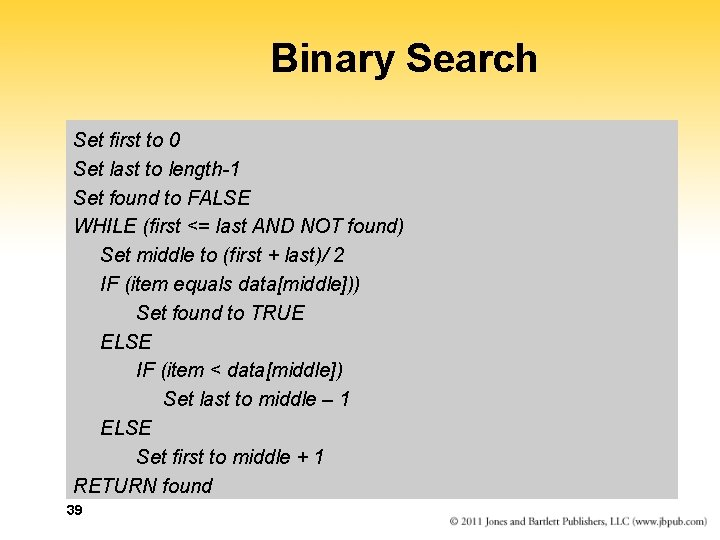Binary Search Set first to 0 Set last to length-1 Set found to FALSE WHILE (first <= last AND NOT found) Set middle to (first + last)/ 2 IF (item equals data[middle])) Set found to TRUE ELSE IF (item < data[middle]) Set last to middle – 1 ELSE Set first to middle + 1 RETURN found 39Binary Search 40 Figure 7. 10 Trace of the binary searchBinary Search Table 7. 1 Average Number of Comparisons 41 Is a binary search always better?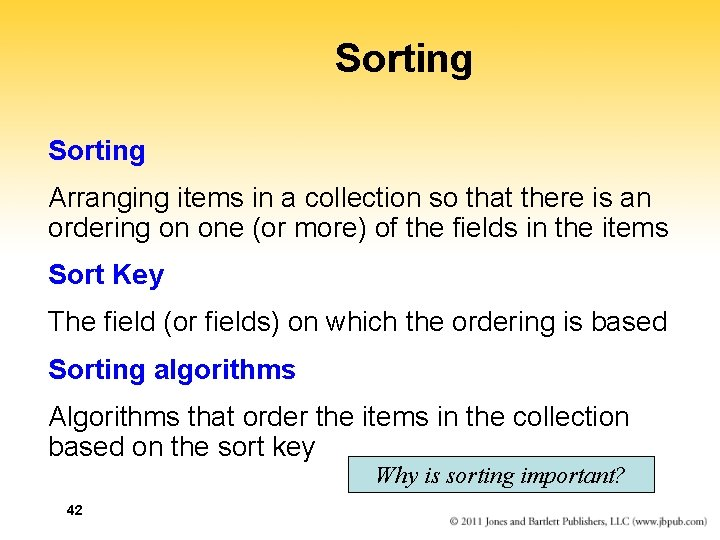Sorting Arranging items in a collection so that there is an ordering on one (or more) of the fields in the items Sort Key The field (or fields) on which the ordering is based Sorting algorithms Algorithms that order the items in the collection based on the sort key Why is sorting important? 42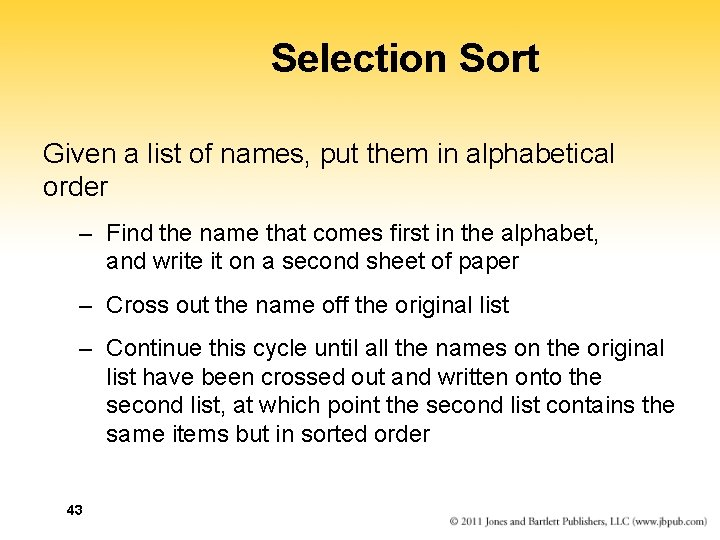Selection Sort Given a list of names, put them in alphabetical order – Find the name that comes first in the alphabet, and write it on a second sheet of paper – Cross out the name off the original list – Continue this cycle until all the names on the original list have been crossed out and written onto the second list, at which point the second list contains the same items but in sorted order 43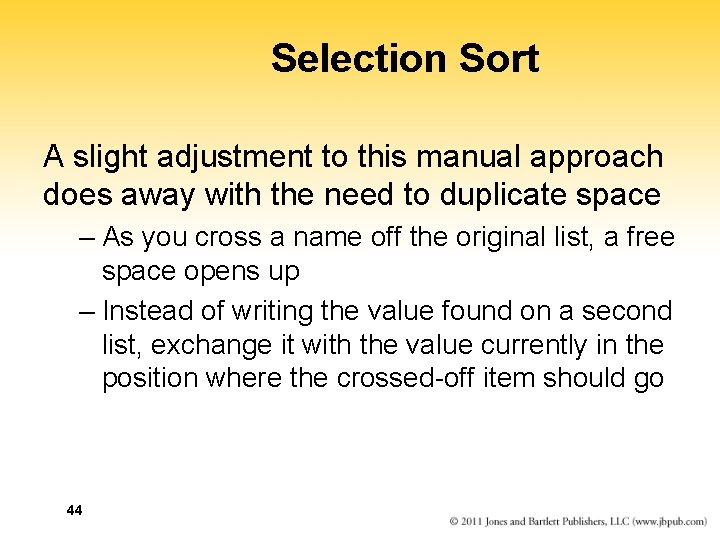Selection Sort A slight adjustment to this manual approach does away with the need to duplicate space – As you cross a name off the original list, a free space opens up – Instead of writing the value found on a second list, exchange it with the value currently in the position where the crossed-off item should go 44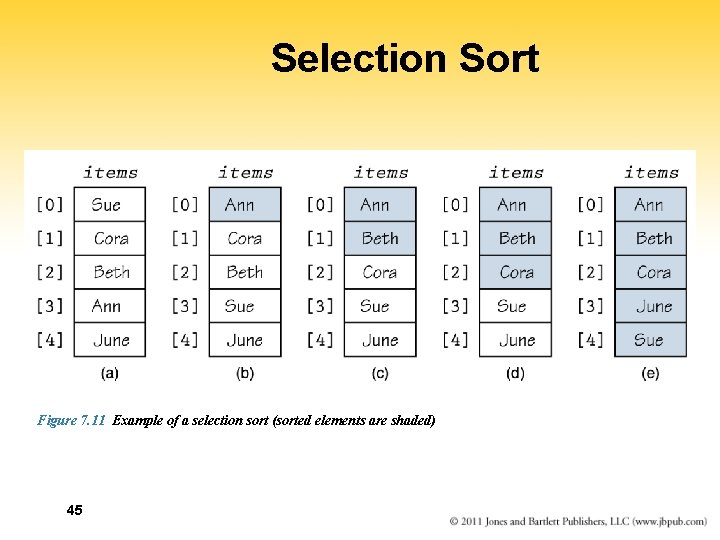Selection Sort Figure 7. 11 Example of a selection sort (sorted elements are shaded) 45Selection Sort Set first. Unsorted to 0 WHILE (not sorted yet) Find smallest unsorted item Swap first. Unsorted item with the smallest Set first. Unsorted to first. Unsorted + 1 Not sorted yet current < length – 1 46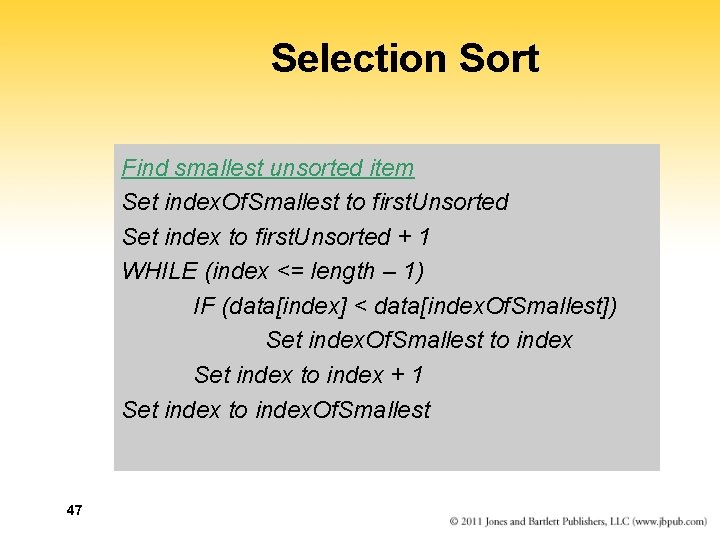Selection Sort Find smallest unsorted item Set index. Of. Smallest to first. Unsorted Set index to first. Unsorted + 1 WHILE (index <= length – 1) IF (data[index] < data[index. Of. Smallest]) Set index. Of. Smallest to index Set index to index + 1 Set index to index. Of. Smallest 47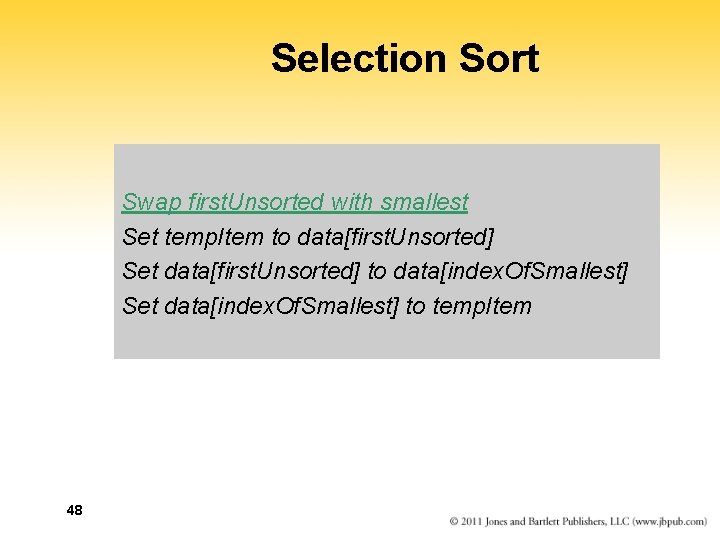Selection Sort Swap first. Unsorted with smallest Set temp. Item to data[first. Unsorted] Set data[first. Unsorted] to data[index. Of. Smallest] Set data[index. Of. Smallest] to temp. Item 48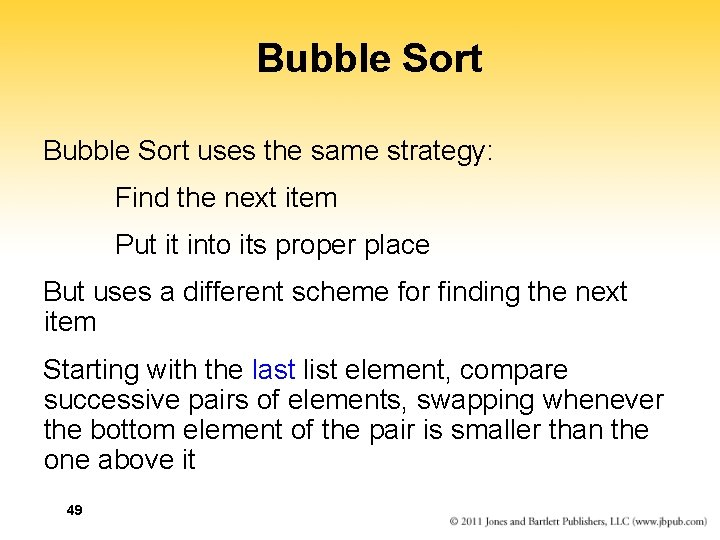Bubble Sort uses the same strategy: Find the next item Put it into its proper place But uses a different scheme for finding the next item Starting with the last list element, compare successive pairs of elements, swapping whenever the bottom element of the pair is smaller than the one above it 49Bubble Sort 50Bubble Sort Bubble sort is very slow! Can you see a way to make it faster? Under what circumstances is bubble sort fast? 51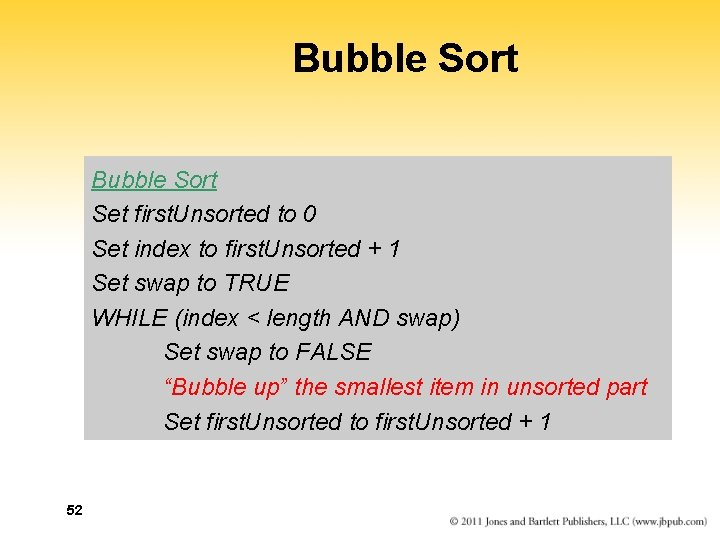Bubble Sort Set first. Unsorted to 0 Set index to first. Unsorted + 1 Set swap to TRUE WHILE (index < length AND swap) Set swap to FALSE “Bubble up” the smallest item in unsorted part Set first. Unsorted to first. Unsorted + 1 52Bubble Sort Bubble up Set index to length – 1 WHILE (index > first. Unsorted + 1) IF (data[index] < data[index – 1]) Swap data[index] and data[index – 1] Set swap to TRUE Set index to index - 1 53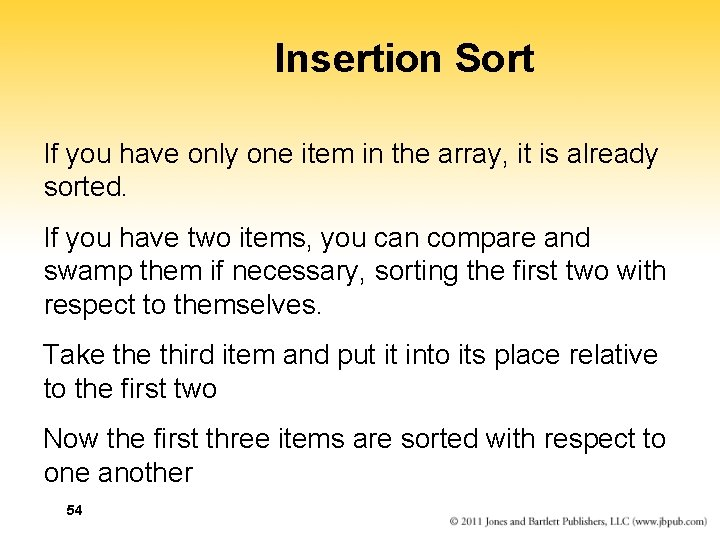Insertion Sort If you have only one item in the array, it is already sorted. If you have two items, you can compare and swamp them if necessary, sorting the first two with respect to themselves. Take third item and put it into its place relative to the first two Now the first three items are sorted with respect to one another 54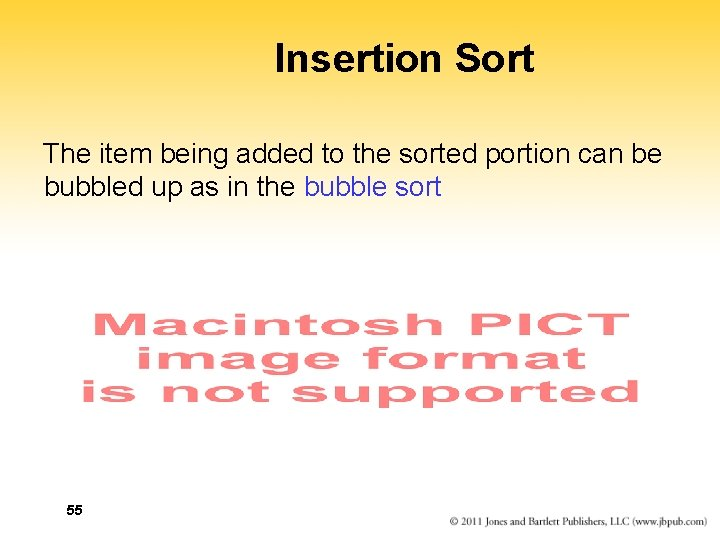Insertion Sort The item being added to the sorted portion can be bubbled up as in the bubble sort 55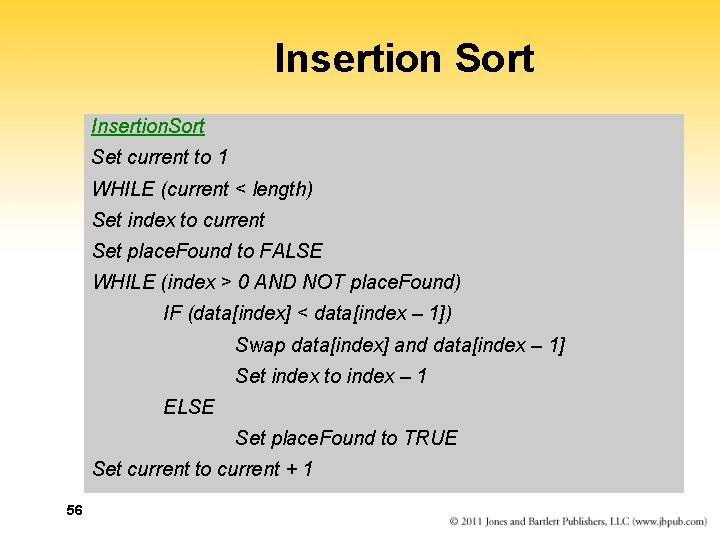Insertion Sort Insertion. Sort Set current to 1 WHILE (current < length) Set index to current Set place. Found to FALSE WHILE (index > 0 AND NOT place. Found) IF (data[index] < data[index – 1]) Swap data[index] and data[index – 1] Set index to index – 1 ELSE Set place. Found to TRUE Set current to current + 1 56Subprogram Statements We can give a section of code a name and use that name as a statement in another part of the program When the name is encountered, the processing in the other part of the program halts while the named code is executed Remember? 57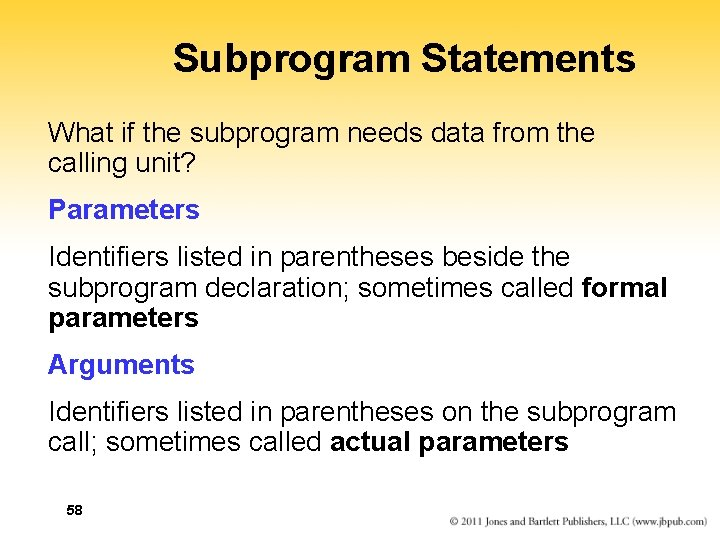Subprogram Statements What if the subprogram needs data from the calling unit? Parameters Identifiers listed in parentheses beside the subprogram declaration; sometimes called formal parameters Arguments Identifiers listed in parentheses on the subprogram call; sometimes called actual parameters 58Subprogram Statements 59 Figure 7. 14 Subprogram flow of control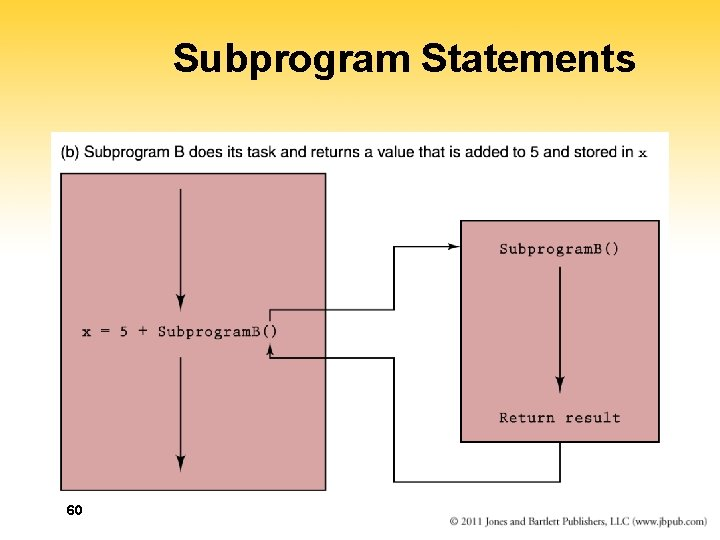Subprogram Statements 60Recursion The ability of a subprogram to call itself Base case The case to which we have an answer General case The case that expresses the solution in terms of a call to itself with a smaller version of the problem 61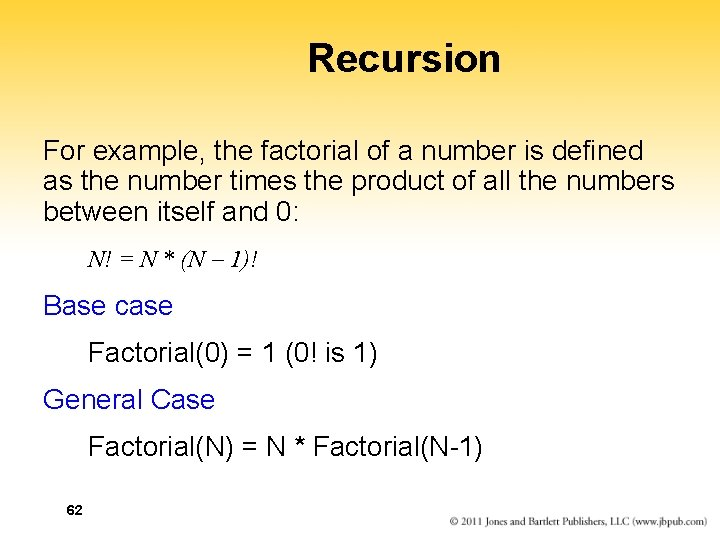Recursion For example, the factorial of a number is defined as the number times the product of all the numbers between itself and 0: N! = N * (N 1)! Base case Factorial(0) = 1 (0! is 1) General Case Factorial(N) = N * Factorial(N-1) 62Recursion Write “Enter n” Read n Set result to Factorial(n) Write result + “ is the factorial of “ + n Factorial(n) IF (n equals 0) RETURN 1 ELSE RETURN n * Factorial(n-1) 63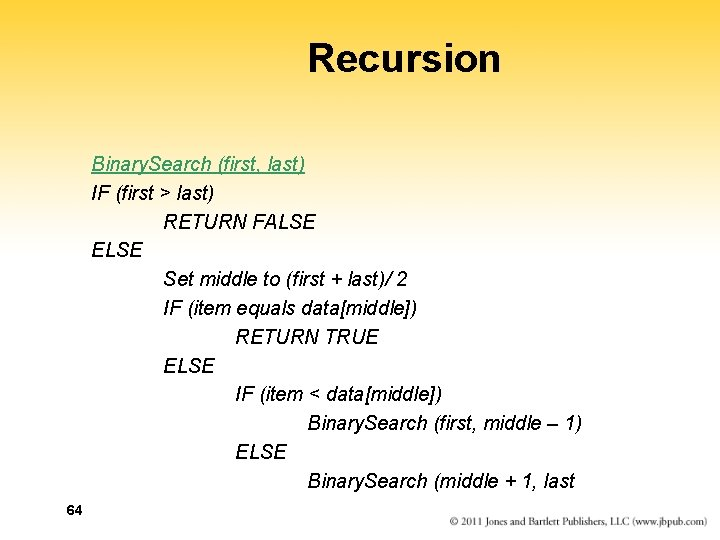Recursion Binary. Search (first, last) IF (first > last) RETURN FALSE ELSE Set middle to (first + last)/ 2 IF (item equals data[middle]) RETURN TRUE ELSE IF (item < data[middle]) Binary. Search (first, middle – 1) ELSE Binary. Search (middle + 1, last 64Quicksort Ordering a list using the Quicksort algorithm 65 It is easier to sort a smaller number of items: Sort A…F, G…L, M…R, and S…Z and A…Z is sortedQuicksort algorithm With each attempt to sort the stack of data elements, the stack is divided at a splitting value, split. Val, and the same approach is used to sort each of the smaller stacks (a smaller case) Process continues until the small stacks do not need to be divided further (the base case) The variables first and last in Quicksort algorithm reflect the part of the array data that is currently being processed 66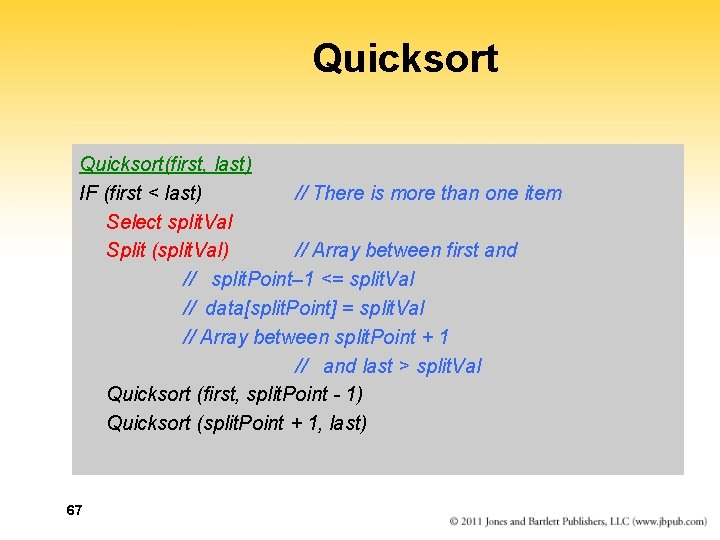Quicksort(first, last) IF (first < last) // There is more than one item Select split. Val Split (split. Val) // Array between first and // split. Point– 1 <= split. Val // data[split. Point] = split. Val // Array between split. Point + 1 // and last > split. Val Quicksort (first, split. Point - 1) Quicksort (split. Point + 1, last) 67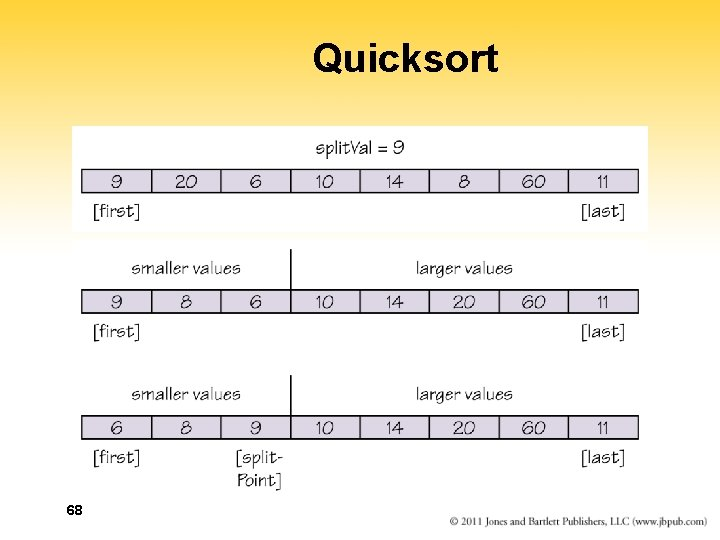Quicksort 68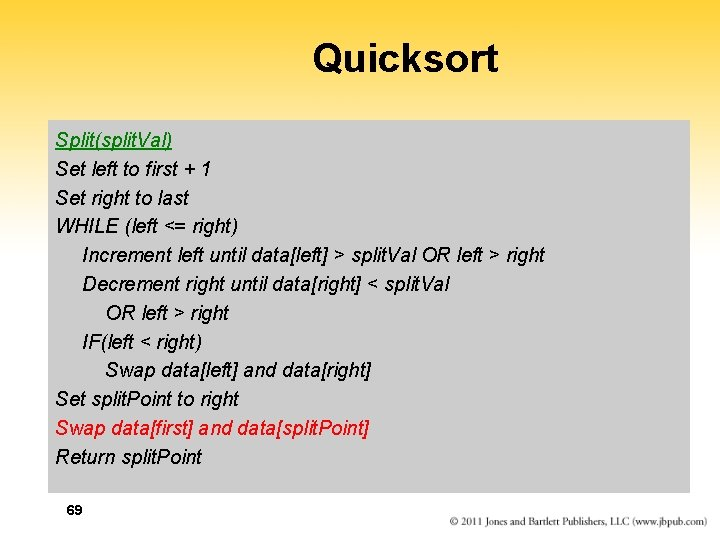Quicksort Split(split. Val) Set left to first + 1 Set right to last WHILE (left <= right) Increment left until data[left] > split. Val OR left > right Decrement right until data[right] < split. Val OR left > right IF(left < right) Swap data[left] and data[right] Set split. Point to right Swap data[first] and data[split. Point] Return split. Point 69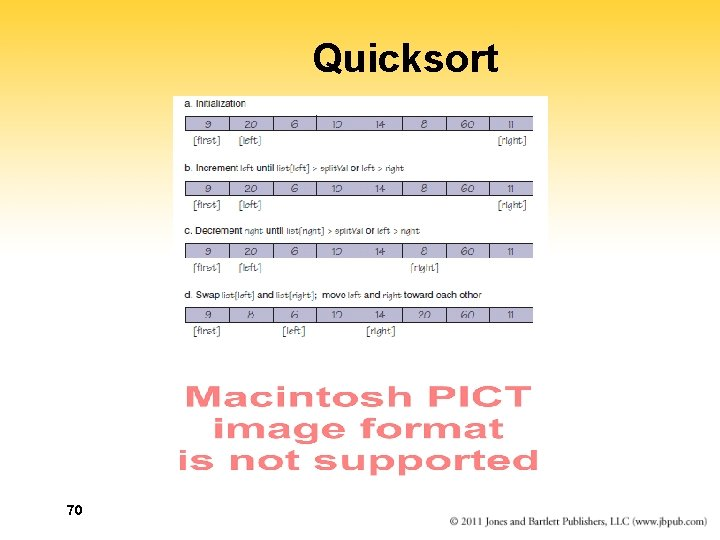Quicksort 70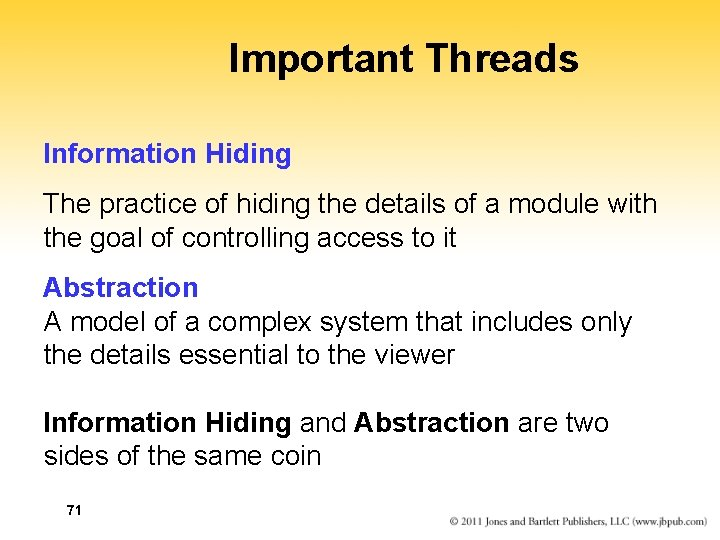Important Threads Information Hiding The practice of hiding the details of a module with the goal of controlling access to it Abstraction A model of a complex system that includes only the details essential to the viewer Information Hiding and Abstraction are two sides of the same coin 71Important Threads Data abstraction Separation of the logical view of data from their implementation Procedural abstraction Separation of the logical view of actions from their implementation Control abstraction Separation of the logical view of a control structure from its implementation 72Important Threads Identifiers Names given to data and actions, by which – we access the data and Read first. Name, Set count to count + 1 – execute the actions Split(split. Val) Giving names to data and actions is a form of abstraction 73